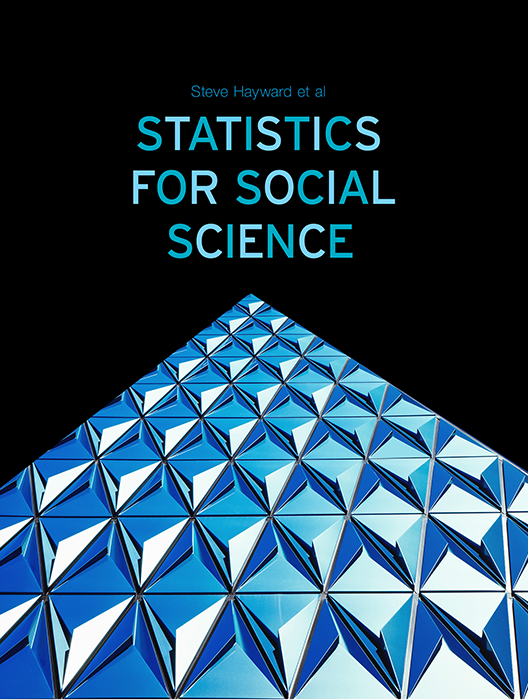# Statistics for Social Science

Lead Author(s): Stephen Hayward

Student Price: Contact us to learn more

Statistics for Social Science takes a fresh approach to the introductory class. With learning check questions, embedded videos and interactive simulations, students engage in active learning as they read. An emphasis on real-world and academic applications help ground the concepts presented. Designed for students taking an introductory statistics course in psychology, sociology or any other social science discipline.

This content has been used by 8,525 students

## What is a Top Hat Textbook?

Top Hat has reimagined the textbook – one that is designed to improve student readership through interactivity, is updated by a community of collaborating professors with the newest information, and accessed online from anywhere, at anytime.

• Top Hat Textbooks are built full of embedded videos, interactive timelines, charts, graphs, and video lessons from the authors themselves
• High-quality and affordable, at a significant fraction in cost vs traditional publisher textbooks

## Key features in this textbook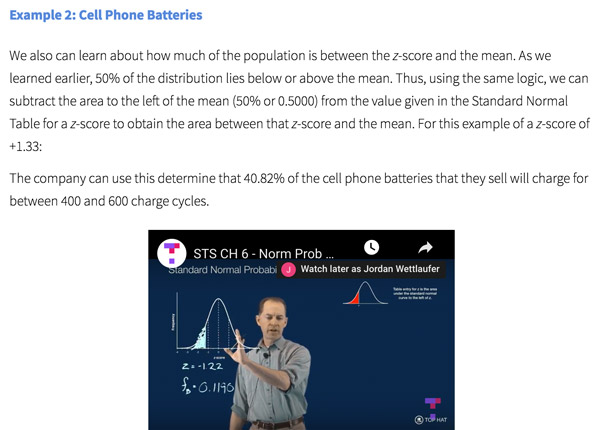Our Statistics for Social Science textbook allows students to manipulate data, visualize the effects discussed, and explore Lightboard videos that feature instructor explanations to reinforce concepts and calculations.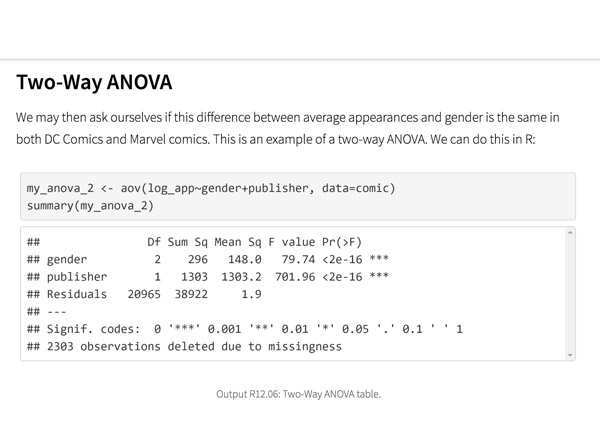Top Hat’s interactive offering includes a complementary module on using R software for data management, graphics, and conducting statistical analyses with examples and practice questions.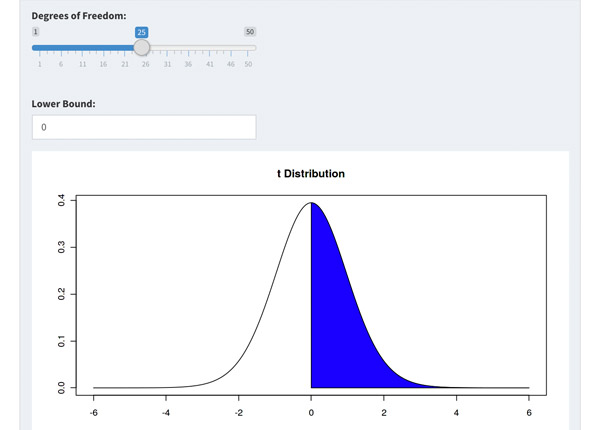Built-in assessment questions embedded throughout chapters so students can read a little, do a little, and test themselves to see what they know!

## Comparison of Social Sciences Textbooks

Consider adding Top Hat’s Statistics for Social Sciences textbook to your upcoming course. We’ve put together a textbook comparison to make it easy for you in your upcoming evaluation.

### Top Hat

Steve Hayward et al., Statistics for Social Sciences, Only one edition needed

### Pearson

Agresti, Statistical Methods for the Social Sciences, 5th Edition

### Cengage

Gravetter et al., Essentials of Statistics for The Behavioral Sciences, 9th Edition

### Sage

Gregory Privitera, Essentials Statistics for the Behavioral Sciences, 2nd Edition

### Pricing

Average price of textbook across most common format

#### Up to40-60%more affordable

Lifetime access on any device

#### $200.83 Hardcover print text only ####$239.95

Hardcover print text only

#### $92 Hardcover print text only ### Always up-to-date content, constantly revised by community of professors Content meets standard for Introduction to Anatomy & Physiology course, and is updated with the latest content ### In-Book Interactivity Includes embedded multi-media files and integrated software to enhance visual presentation of concepts directly in textbook Only available with supplementary resources at additional cost Only available with supplementary resources at additional cost Only available with supplementary resources at additional cost ### Customizable Ability to revise, adjust and adapt content to meet needs of course and instructor ### All-in-one Platform Access to additional questions, test banks, and slides available within one platform ## Pricing Average price of textbook across most common format ### Top Hat Steve Hayward et al., Statistics for Social Sciences, Only one edition needed #### Up to40-60%more affordable Lifetime access on any device ### Pearson Agresti, Statistical Methods for the Social Sciences, 5th Edition ####$200.83

Hardcover print text only

### Pearson

Gravetter et al., Essentials of Statistics for The Behavioral Sciences, 9th Edition

#### $239.95 Hardcover print text only ### Sage McConnell, Brue, Flynn, Principles of Microeconomics, 7th Edition ####$92

Hardcover print text only

## Always up-to-date content, constantly revised by community of professors

Constantly revised and updated by a community of professors with the latest content

### Top Hat

Steve Hayward et al., Statistics for Social Sciences, Only one edition needed

### Pearson

Agresti, Statistical Methods for the Social Sciences, 5th Edition

### Pearson

Gravetter et al., Essentials of Statistics for The Behavioral Sciences, 9th Edition

### Sage

Gregory Privitera, Essentials Statistics for the Behavioral Sciences, 2nd Edition

## In-book Interactivity

Includes embedded multi-media files and integrated software to enhance visual presentation of concepts directly in textbook

### Top Hat

Steve Hayward et al., Statistics for Social Sciences, Only one edition needed

### Pearson

Agresti, Statistical Methods for the Social Sciences, 5th Edition

### Pearson

Gravetter et al., Essentials of Statistics for The Behavioral Sciences, 9th Edition

### Sage

Gregory Privitera, Essentials Statistics for the Behavioral Sciences, 2nd Edition

## Customizable

Ability to revise, adjust and adapt content to meet needs of course and instructor

### Top Hat

Steve Hayward et al., Statistics for Social Sciences, Only one edition needed

### Pearson

Agresti, Statistical Methods for the Social Sciences, 5th Edition

### Pearson

Gravetter et al., Essentials of Statistics for The Behavioral Sciences, 9th Edition

### Sage

Gregory Privitera, Essentials Statistics for the Behavioral Sciences, 2nd Edition

## All-in-one Platform

Access to additional questions, test banks, and slides available within one platform

### Top Hat

Steve Hayward et al., Statistics for Social Sciences, Only one edition needed

### Pearson

Agresti, Statistical Methods for the Social Sciences, 5th Edition

### Pearson

Gravetter et al., Essentials of Statistics for The Behavioral Sciences, 9th Edition

### Sage

Gregory Privitera, Essentials Statistics for the Behavioral Sciences, 2nd Edition

## About this textbook

### Lead Authors

#### Steve HaywardRio Salado College

A lifelong learner, Steve focused on statistics and research methodology during his graduate training at the University of New Mexico. He later founded and served as CEO of Center for Performance Technology, providing instructional design and training development support to larger client organizations throughout the United States. Steve is presently lead faculty member for statistics at Rio Salado College in Tempe, Arizona.

#### Joseph F. Crivello, PhDUniversity of Connecticut

Joseph Crivello has taught Anatomy & Physiology for over 34 years, and is currently a Teaching Fellow and Premedical Advisor of the HMMI/Hemsley Summer Teaching Institute.

## Explore this textbook

Read the fully unlocked textbook below, and if you’re interested in learning more, get in touch to see how you can use this textbook in your course today.

# Introduction to Probability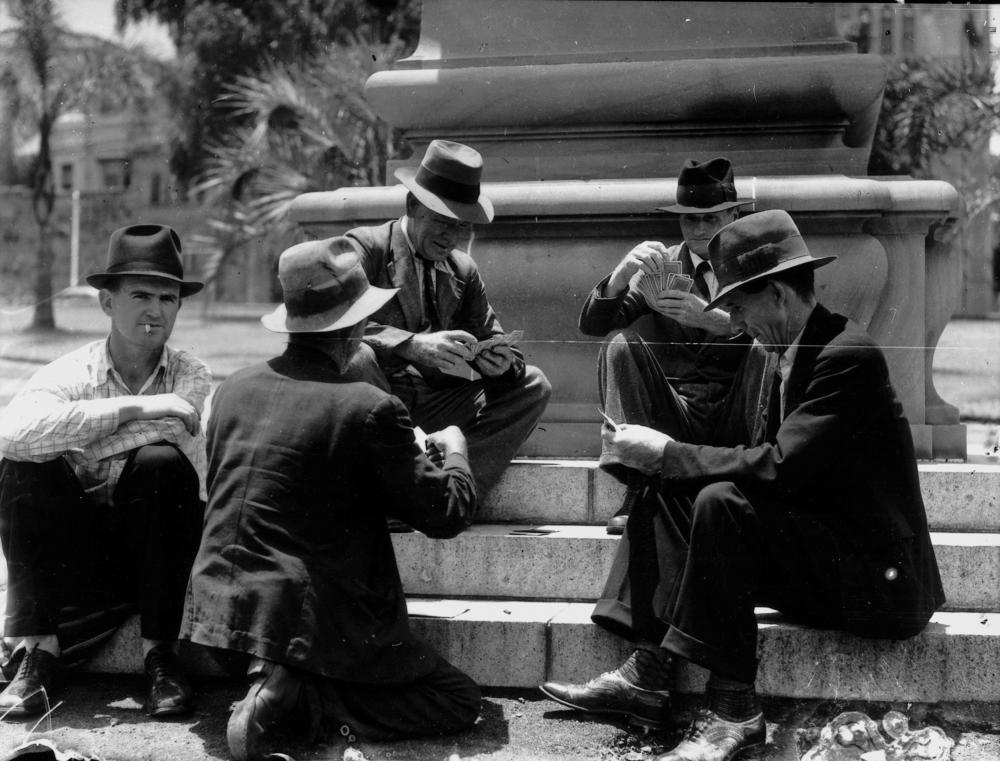Men playing cards in Brisbane, Australia. Probability plays a major role in common card games. ​

## Chapter Objectives

After completing this chapter, you will be able to:

• Identify the sample space of a probability event.
• Distinguish among classical probability, empirical probability, and subjective probability.
• Find the probability of an event given that another event has occurred.
• Distinguish between independent and dependent events.
• Determine if two events are mutually exclusive.
• Use the multiplication rule to find the probability that two events occur in sequence.
• Use the addition rule to find the probability that either of two events occurs.

## Why Study Probability?

We are all familiar with probability, whether or not we actually think of it in scientific terms. We hear a weather forecast, we wonder what the commute to work or school will be like today, or we might wonder what the chances are of getting an A on that upcoming statistics exam! In a very general, but also a very real way, it affects decisions you make on a daily basis. It gives us a way to evaluate the risks associated with just about any sort of endeavor or behavior.

Probability is the key to assessing and understanding the risks involved in any decision-making process, whether in a manufacturing plant, a government agency, or in just about any process involving research and the reporting of outcomes. Social scientists use probability to assess the likelihood (risk) of making incorrect inferences when generalizing about human behavior. Medical researchers must establish the likelihood that a drug or treatment regimen will be effective. Pollsters must be able to assess the accuracy of their findings. The list goes on. Here's an interesting video on whether or not political polls in America should be trusted:

Join Spencer Greenberg of Columbia University’s School of Engineering as he provides some additional insights into how probability is used in real life. His views and insights are guaranteed to get you thinking!

## What is Probability?

At its most basic level, probability can be defined as the likelihood of an event expressed in numerical terms as a fraction, decimal, or percent. A probability of zero indicates the event will never happen (or can’t happen), while a probability of one indicates it will absolutely happen. The range of values for any probability statement, then, can be from 0 to 1. You will often see probabilities expressed as either fractions or decimals. This can be summarized in a single expression as

Examples

If a fair (meaning not biased) die is rolled, the probability of it coming up a six can be expressed as 1/6 since there are six sides to the die and only one side at a time can be “up.”

A fair coin has two sides, heads and tails. The probability of flipping a coin and getting “heads” is ½, or .5. The coin has two sides and it must end up on one side or the other (assuming it cannot land on its edge).

4.01

Probability is key to assessing

A

the amount of error.

B

the likelihood of an event.

C

whether an event occurred by chance.

4.02

A "sure thing" would have a probability equal to $\_\_\_\_$.

4.03

Which value below could not be a probability?

A

.54

B

0

C

.12

D

-.12

## What is a Probability Experiment?

A probability experiment can be defined as a situation involving chance or probability that leads to observable and measurable results called outcomes. A probability experiment could consist of one or many trials where each trial produces one outcome. It could be a very simple experiment or it could be much more complex, involving numerous trials and several series of outcomes. Regardless of the complexity, all probability experiments have three things in common:

• The experiment must have more than one possible outcome.
• Each possible outcome can be specified in advance.
• The outcome of the experiment is due to chance.

Examples

• A die is rolled once to see if the number four comes up (probability 1 out of 6, or approximately .167).
• A coin is tossed to see if a heads occurs (probability 1 out of 2, or .5).
• A coin is tossed four times to measure how many heads come up (probability 1 out of 2 to the 4th power, or .54).

Non-Examples

A non-example of a probability experiment is something that has only one possible outcome, or for which the possible outcomes cannot be specified in advance, or the outcomes are not due to chance.

• “A football player runs for a touchdown” is not a probability experiment.
• “A person buys a raffle ticket” is not a probability experiment.
• “Your church sponsors a Las Vegas night to raise money for charity” is not a probability experiment.

### What is a Trial?

A trial can be defined as a single performance of a probability experiment.

Examples

• In the die rolling experiment, one roll of the die represents one trial.
• In the first coin toss experiment, the coin toss represents one trial.
• In the second coin toss experiment, the series of four tosses represents one trial.

### What is an Outcome?

An outcome is the result of a single trial of a probability experiment.

Examples

• In the die rolling experiment, the result of that roll of the die represents the outcome.  There are six possible outcomes, 1 through 6.
• In the first coin toss experiment, the result of that toss represents the outcome. There are two possible outcomes, H or T.
• In the second coin toss experiment, the result of one series of tosses represents the outcome of that trial. There are 16 possible outcomes, shown in the examples below.

### What is a Set?

Set notation is used in probability to describe collections of objects or values that have been defined according to a rule or statement. Each object or value included in the set is an element of that set. Sets are often denoted by a capital letter, e.g., A, B, or C. The objects or values that comprise the set are enclosed in braces.

Examples

In the die rolling experiment, the set of all possible outcomes of that trial is represented as {1, 2, 3, 4, 5, 6}, since any number from 1 to 6 is a possible outcome. Each number from 1 to 6 is an element of the set.

Sets of outcomes will be explored more in the section on sample space, below.

### What is an Event?

A formal definition of an event in statistics is a subset of the sample space, which in turn is a collection of all possible outcomes of a probability experiment. An event, then, can consist of one or more outcomes that satisfy a given condition and can be either simple or compound.

• A simple event consists of a single outcome.
• A compound event consists of more than one outcome.

Example

A die is rolled once to see if the number four comes up. The roll of the die represents one trial. The result of that roll of the die represents the outcome. The set of all possible outcomes of that trial is represented as {1, 2, 3, 4, 5, 6} since any number from 1 – 6 is a possible outcome.

• The event, rolling a four, has only one possible outcome: {4}. It is a simple event.
• The event, rolling a number that is not a four, has five possible outcomes: {1, 2, 3, 5, 6}. It is a compound event.

4.04

Which of the following is NOT a probability experiment?

A

Wagering on the outcome of a spin of a roulette wheel

B

Guessing the answer to a multiple choice quiz question

C

A soccer player scoring a goal

D

Selecting a square on the office football pool

4.05

The probability experiment of rolling a single die to see if a number greater than 2 comes up has how many outcomes?

4.06

In a probability experiment involving the roll of a die, the event “rolling a number greater than five” is a $\_\_\_\_\_\_\_\_$ event.

## What is a Sample Space?

A sample space is the description of all possible outcomes of a probability experiment. Writing out the description of a sample space is often the first step in determining the probability of any single outcome. The sum of the probabilities of all of the possible outcomes in the sample space must be equal to 1. Therefore, any given outcome can be represented by the random variable x and its probability calculated as:

Depending on the complexity of the experiment, there are several ways a sample space can be summarized and presented. Examples of different sample spaces are presented below.

### Describing the Sample Space with a Set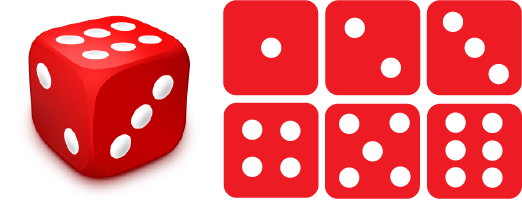Figure 4.1: Set of outcomes from rolling a die​

The sample space of the probability experiment of rolling a die would include six possible outcomes, rolling a 1 through a 6. It is shown as:

{1, 2, 3, 4, 5, 6}

The braces enclosing the outcomes indicate this is a set of values.

### Describing the Sample Space with a Tree Diagram

A tree diagram is also a useful tool to help communicate the outcomes of a probability experiment. A probability experiment consisting of first flipping a coin, then, if a head occurs, flipping the coin a second time, but if a tail occurs, tossing a die, is a bit complicated to describe in words, but is easily seen in a tree diagram.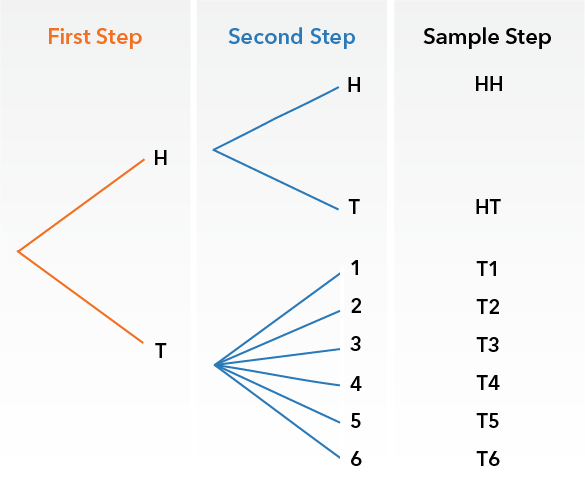Figure 4.2: Tree diagram of a probability experiment​

### Describing the Sample Space with a Table

A previous example described a probability experiment of tossing a fair coin four times to measure how many times it comes up heads. Since the coin is fair, meaning unbiased, we know there is an equal chance of either heads or tails coming up on any toss. The coin tosses constitute a probability experiment, and the sample space summarizes all the possible outcomes of that experiment.

The sample space can be defined as:

S = {(HHHH), (HHHT), (HHTH), (HTHH), (THHH), (HHTT), (HTTH), (HTHT), (TTHH), (THHT), (THTH), (TTTH), (TTHT), (THTT), (HTTT), (TTTT)}

All possible outcomes of the coin toss experiment can also be summarized in a chart of the sample space as: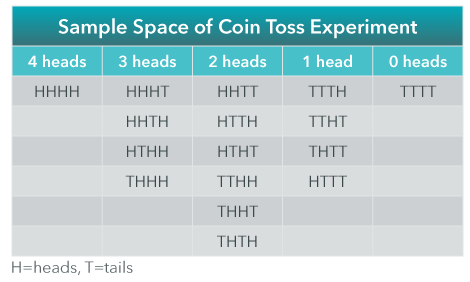Figure 4.3: Sample space of coin toss​

## Events

As you can see in the chart, there are 16 possible outcomes of the experiment. Once all possible outcomes are described, the probability of the events of interest (the outcomes of “heads”) can be easily calculated.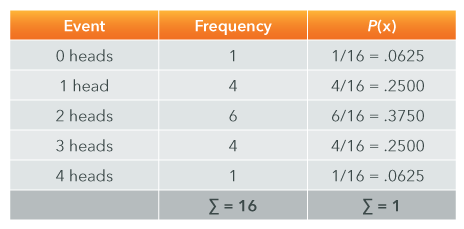Figure 4.4: Summary of outcomes​​

The probabilities associated with the events in the table above can be displayed graphically as in the chart below. Notice how closely it approximates a normal distribution!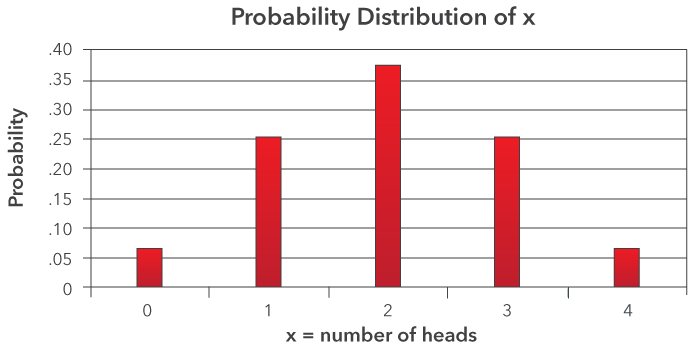Figure 4.5: Probability distribution of x​​​

### Complements of Events

The complement of an event is all outcomes that are not the event. The complement of Event A is the event not occurring. It is designated as A’ (A prime). The relationship can be shown asFigure 4.6: Coin toss​

If Event A in the probability experiment of tossing a coin four times is the event of one head, we can see from the sample space that P(A) = 4/16, or .25.

In that case, the complement of Event A would be all outcomes that are not one head, which is all remaining outcomes in the sample space. The probability associated with A’ can be calculated as:

P(A’) = 1 – P(A) = 1 - .25 = .75

4.07

One marble is drawn from a jar containing 4 red, 6 blue, and 7 green marbles. What is the sample space?

A

{4, 6, 7}

B

4, 6, 7

C

{red, blue, green}

D

{4 red, 6 blue, 7 green}

4.08

In the sample space for the probability experiment of rolling a pair of dice, how many outcomes are there?

4.09

In a probability experiment, the set of all possible outcomes represents the sample space. A subset of those outcomes represents a(n) $\_\_\_\_\_\_\_\_\_\_$.

## Types of Probability

There are three ways to arrive at a probability statement, each based on an approach to the probability in question. They are:

• Theoretical probability
• Empirical probability
• Subjective probability

### Theoretical Probability

Theoretical probability, or classical probability, is based on a theoretical assumption about the nature of the event, in which it is assumed that n events are equally likely to occur. For example, the theoretical probability of rolling a two on a six-sided die is one-sixth.

### Empirical Probability

Empirical probability, or relative frequency probability, is based on the observed outcomes of one or a series of trials. It is the type used most often in statistical inference procedures. For example, a die is rolled 10 times and the number two comes up two times, making the relative frequency 2/10.

### Subjective Probability

Subjective probability is based on an individual’s personal belief about the likelihood of an event occurring. Since it is based on personal beliefs it is often different from others’ subjective probability of the same event(s). It is also not based on any real math or formal calculations and is therefore unreliable. For example, “the probability of winning the lottery is .5” may not accurately reflect the reality of the situation.Figure 4.7: Probability types ​

Example: Theoretical Probability

A graduate student is setting up an experiment using laboratory rats to investigate the effect of rewards on learning. In one part of the experiment, the rats are released into a maze with four corridors, one of which leads to a bar-press mechanism where the rat can obtain a food pellet. Assuming that on the first trial there is an equal likelihood of choosing any of the four corridors, what is the probability the rat will choose the corridor leading to the food reward (labeled as corridor D)?

In this case the event (E) = choosing corridor D. There is one event that would be a success, and three events that would not be successes. There is one outcome in event E, and the total number of outcomes in the sample space is four. So, P(E) = P(D) = 1/4 = .25. You can see this in the sample space:Figure 4.8: Corridor choices

If the rat is placed in the middle of a circular maze, then each outcome is equally likely. As a result, the sum of the probabilities can be shown as P(A) + P(B) + P(C) + P(D) = .25 + .25 + .25 + .25 = 1. The probabilities sum to 1.0 because one of them must occur; the only question is which one?

Example: Empirical Probability

A school psychologist is interested in the number of children that families in a certain town have enrolled in elementary school. She sends out 1000 surveys, of which 800 are returned. Of those 800, the data reported are: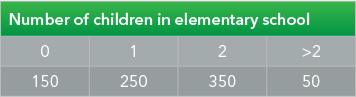Figure 4.9: Children in elementary school ​

Based on these data, the psychologist could conclude that the probability of a family in that town having two children enrolled in elementary school is P(E) = P(2) = 350/800 = .4375.

Examples: Subjective Probability

1. During a classroom discussion of political issues, one student states that Donald Trump “has a 50% chance of being re-elected.” This is an example of subjective probability since it is based on the student’s personal opinion and cannot be verified empirically.

2. Lotteries have been around for ages, but have more recently become highly sophisticated vehicles for raising funds for a variety of causes and agencies. Alice walks into a convenience store to purchase a lottery ticket. She thinks, in a rather offhand way, that her chance of winning one of the prizes might be as low as one in a hundred but is willing to invest a couple of dollars anyway, since it is just “loose change.”

Based on this subjective estimate, P(E) = P(winning) = .01.

The complement of this event, not winning the lottery, would be equal to 1 - .01 = .99.

4.10

Match the following examples with a type of probability.

Premise
Response
1

A die is rolled ten times and the number of threes recorded.

A

Theoretical

2

The likelihood of a coin coming up heads.

B

Empirical

3

The Yankees chances of winning the series.

C

Subjective

## Types of Events

There are four types of events in probability experiments that we need to know about. They are:

• Independent events
• Dependent events
• Mutually exclusive events
• Complementary events

### Independent Events

If two events are independent, the probability that one will occur is not affected by whether or not the other has occurred.

Examples

• If a die is rolled twice (two trials) and the numbers recorded, the outcome of the second trial is not affected by the outcome of the first trial. The probability of any number from one to six coming up on either trial is one-sixth.
• It is unlikely that the probability of a student registering to take Introductory Psychology and the probability of having Cheerios for breakfast are related in any meaningful way, so it is probably safe to assume they are independent events.

### Dependent Events

If two events are dependent, the probability that one will occur is affected (changed) by the occurrence of the other.

Examples

• If a queen is drawn from a deck of cards on the first trial and is not replaced, the probability of drawing another queen on the second trial is changed as a result of the outcome of the first trial. Prior to the first trial, there were four queens in the deck, but after one was removed there were only three available for the second trial. The probability of drawing a queen changed from 4/52 on the first trial to 3/51 on the second trial.
• Health psychologists have related behavioral risk factors to health-related outcomes. One such is excessive consumption of alcohol, which has been found to be related to an increased incidence of liver cancer. Alcohol consumption and the development of liver cancer are, in that respect, dependent events since the probability of developing liver cancer is affected (changed) by the occurrence of excessive alcohol consumption. Note that this is not to say that liver cancer will develop as a result of excessive alcohol consumption, only that the probability is affected by the prior behavior.

4.11

Match the following examples with a type of event.

Premise
Response
1

Cigarette smoking and developing lung cancer

A

Dependent

2

Rolling “snake eyes” after rolling a string of sevens

B

Independent

### Mutually Exclusive Events

Two events are mutually exclusive if they cannot occur at the same time. Cramming for an exam and training for a bicycle race are mutually exclusive events. You might be doing one or the other but not both at the same time. Pumping gas into your car and driving are mutually exclusive events that are also dependent (presuming you have a gasoline-powered vehicle).

Mutually exclusive and not mutually exclusive events can be represented graphically using Venn diagrams. Venn diagrams, a.k.a. set diagrams, are used to show all possible logical relations between sets of items. If you’d like to experiment with the use of Venn diagrams, Academo.org maintains a website with a Venn diagram interactive tool that is available here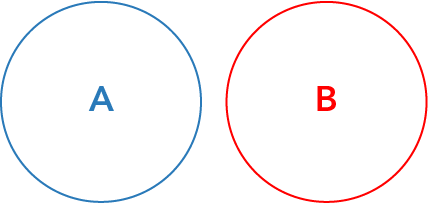Figure 4.10: Venn diagram of mutually exclusive events​

The two events A and B represented by the circles above are mutually exclusive. The circles do not overlap, indicating there are no shared attributes or elements. This may also be shown as P(A ∩ B) = 0, meaning the probability of the intersection of events A and B is zero.

Note: “Intersection” is the event where both A and B would occur. The “upside down U” is the symbol indicating an intersection.

Suppose two events have a non-zero chance of occurring. Then, if the two events are mutually exclusive, they can not be independent. If two events are independent, they cannot be mutually exclusive.

Examples: Mutually Exclusive Events

• Being a dog or a cat are events that are mutually exclusive. A pet cannot be both a dog and a cat at the same time.Figure 4.11: Venn diagram of pets​
• Selecting a queen from a card deck, not replacing it, and then selecting a king are dependent events that are mutually exclusive. They are mutually exclusive because you cannot draw both a king and a queen at the same time and are dependent since the initial withdrawing of a queen from the deck affects the probability of drawing a king on the next draw. Note that if the queen had been replaced in the deck prior to making the second draw the events would not be dependent.Figure 4.12: Venn diagram of card selection​

### Not Mutually Exclusive Events

Two events are not mutually exclusive if they can occur at the same time. One event could be a subset of the other (complete overlap), or the events may have some proportion of shared elements. This can be shown graphically by Venn diagrams in which a portion of the representative circles overlaps to show an intersection.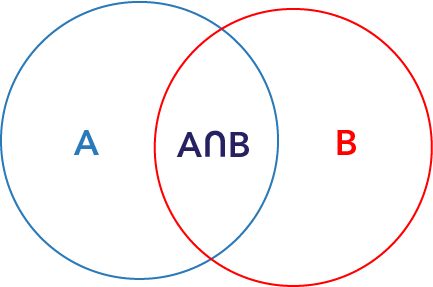Figure 4.13: Venn diagram of not mutually exclusive events​

These two events, represented by the circles above, are not mutually exclusive. They have shared elements that are common to both A and B, represented by the intersection of A and B.

Examples: Mutually Exclusive vs. Not Mutually Exclusive Events

Not Mutually Exclusive Example

In a group of students, 40 are juniors, 50 are English majors and 22 are English-major juniors. The 22 English juniors represent elements shared by the two categories “juniors” and “English majors” and could be represented graphically as the intersection of those categories. Since the categories have shared elements, they are not mutually exclusive. A student can be both an English major and a junior.Figure 4.14: Venn diagram of juniors and English majors​

Mutually Exclusive Example

In another group of students, there are 30 juniors and 35 seniors. At least in terms of class ranking, there are no shared elements, so these categories are mutually exclusive. A student cannot be both a junior and a senior.Figure 4.15: Venn diagram of junior and senior students​

### Complementary Events

Complementary events are two outcomes of a trial that are the only two possible outcomes. One event occurs if and only if the other event does not occur. This also implies that the events are mutually exclusive since only one or the other of the two outcomes can occur.

Examples

• Flipping a coin and getting heads or tails are complementary outcomes because there are no other outcomes possible; the result must be one or the other.
• On the other hand, rolling a die and getting either a one or two are not complementary outcomes because there are other possible outcomes, like rolling a 3, 4, 5, or 6.

This illustrates that all complementary events are mutually exclusive, but all mutually exclusive events are not necessarily complementary!

4.12

Identify the true statement.

A

Events that are not mutually exclusive are also not complementary

B

Events with shared elements are mutually exclusive

C

Mutually exclusive events are also not complementary

D

Dependent events are also not mutually exclusive

4.13

The events "getting a final grade of A in statistics" and "getting a final grade of C in statistics" are _________.

A

Complementary events

B

Dependent events

C

Mutually exclusive

D

Not mutually exclusive

4.14

Two $\_\_\_\_\_\_\_$ events, when combined, make up the entire sample space.

## The Fundamental Counting Principle

The fundamental counting principle is a mathematical rule that enables us to find the number of ways a combination or series of independent events can occur. Among other things, it can be used to quickly determine the total possible outcomes in a sample space without having to create a diagram. It states that if there are a ways for one outcome or event to occur, and b ways for a second to occur, then there are (a × b) ways for both to occur.

This can be extended to multiple outcomes or events. If there are a ways for one to occur, b ways for a second, and c ways for a third, there are (a × b × c) ways for all three to occur.

Examples

• In a probability experiment to flip a coin and roll a die, the sample space can be expressed as S = {H1, H2, H3, H4, H5, H6, T1, T2, T3, T4, T5, T6}. We can count the outcomes to see that they total 12 possible outcomes or, instead of diagraming and counting, we could simply have calculated the total number of ways using the fundamental counting principle. We know there are two possible outcomes to a coin flip and six possible outcomes from rolling a die, so the total possible outcomes can be calculated as (2 × 6) = 12.
• In the probability experiment of tossing a coin four times, the number of possible outcomes could be calculated as (2 × 2 × 2 × 2) = 24 = 16. That was a whole lot quicker than creating the set or the table we used earlier (but maybe not as much fun?).

4.15

The access code for a security door consists of four digits. Each digit can be any number from 0 through 9. How many access codes are possible?

## The Multiplication Rule

The multiplication rule can be used to find the probability that two (or more) events occur in sequence and takes the fundamental counting principle a step further by taking into account both independent and dependent events. The process changes a bit, depending on whether the events are independent or dependent, and we’ll address each of those in turn.

It might be useful to think of this as the probability of one event and another event, i.e., probability “and.”

### Independent Events

If events are independent, the probability that they will all occur is equal to the product of their individual probabilities. (The product is the result of multiplying two or more numbers.) This is the most basic application of the multiplication rule, and can be stated as:

Examples

• Rolling a die and making a selection from a deck of cards are independent events since the occurrence of either event has no effect on the probability associated with the other event. We could, then, apply the multiplication rule shown above to determine the probability of rolling a die and having a six come up and drawing a card and getting a spade. The probabilities can be represented as:

The probability of rolling a six and then drawing a spade is 1/24.

• A recent campus poll concluded that 10 out of 100 students at the school prefer pizza for dinner. If we were to have a computer program select one student at random from the student body, the probability of selecting a student who prefers pizza for dinner would be .10. If we let this represent event A, then P(A) = .10.

The Wechsler Adult Intelligence Scale (WAIS) is a standardized test of intellectual ability, arguably measuring intelligence. Based on normative data collected from the general population, it has been determined to have a mean of 100 and a standard deviation of 15. The probability of a person selected at random scoring >115 (one S.D. above the mean) is, according to the Empirical Rule, approximately .16 (check this, if you’re not sure how that is determined, by reviewing the empirical rule). If we let this represent event B, then P(B) = .16. If we then wanted to determine the overall probability of selecting a student who prefers pizza for dinner and who also scored more than 115 on the WAIS (assuming the distribution of WAIS scores is the same as for the general population), the equation looks like this:​

The probability that both of these events would occur is approximately .016.

### Dependent Events and Conditional Probability

If two events are dependent, we know that the probability that one will occur is affected by whether or not the other has occurred. This is conditional probability. It can be summarized as the probability of event B occurring, given that event A has occurred. It is stated as the probability of B, given A, and is represented as P(B | A).

To find the probability that two events, one of which is dependent on the other, will occur in sequence involves three steps: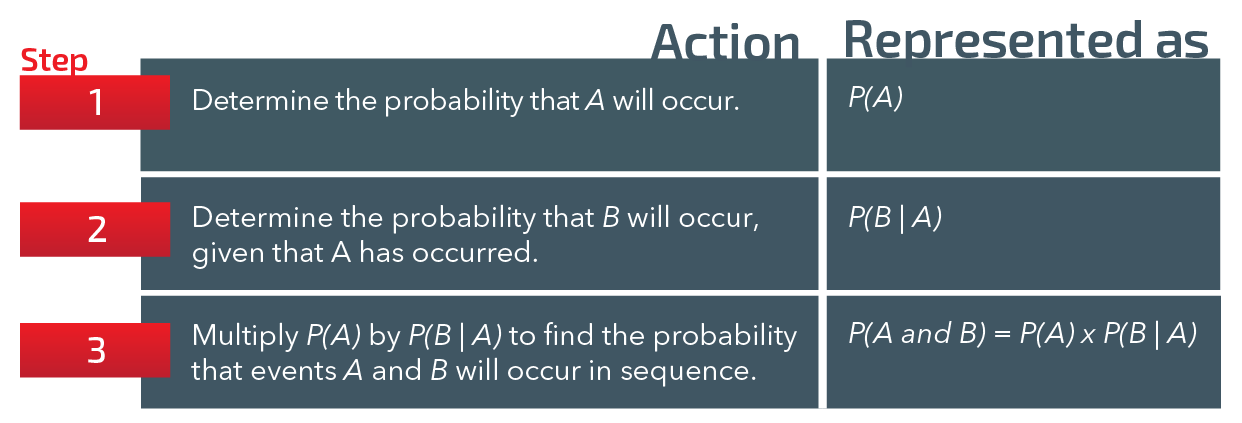Figure 4.16: Procedure table for multiplication rule

Examples

• There are ten marbles—three blue, three red, and four green—in a bag. To find the probability of randomly selecting a blue marble after a green has already been selected and removed from the bag, we could use the formula for conditional probability, since these are dependent events (the probability of selecting blue changes as a result of the green having been removed). Following the steps above:

Step 1: P(A) = selecting a green marble = 4/10

Step 2: P(B | A) = selecting a blue marble after selecting a green = 3/9 = 1/3

Step 3: P(A and B) = P(A) × P(B | A) = 4/10 × 1/3 = 4/30 = 2/15

The probability of selecting a green marble followed by selecting a blue marble is 2/15.

• The probability that a psychology major will take Introduction to Statistics is approximately .9 (since most psychology programs require a course in statistics). Of students who have completed an introductory statistics course, approximately 25% will go on to take a more advanced course, Research Methods. Introduction to Statistics is a prerequisite for Research Methods.

The psychology department chair would like to determine the probability that a psychology major will take Research Methods, and has created the steps below to guide her.

Step 1: P(A) = .9, the probability that a psychology major will take Introduction to Statistics.

Step 2: P(B | A) = .25, the probability that a psychology major who has completed Introduction to Statistics will go on to take Research Methods.

Step 3: P(A and B) = P(A) x P(B | A), = .9 x .25 = .225, the probability that a psychology major will take Research Methods.

4.16

According to data from the American Cancer Society, about 1 in 3 women living in the U.S. will have some form of cancer during their lives. If three women are randomly selected, what is the probability that they will all contract cancer at some point during their lives?

A

1/81

B

1/27

C

1/3

4.17

85% of the students at a certain community college have graduated. 35% of those graduates go on to complete bachelor’s degrees. What is the probability that a student drawn at random will complete a bachelor’s degree?

4.18

The probability of event B given that event A has occurred is known as $\_\_\_\_\_\_$ probability.

## The Addition Rule

The addition rule can be used to find the probability that at least one of two events will occur. Note how this differs from the multiplication rule: the multiplication rule is used to find the probability that both (or all) events will occur, while the addition rule is used to find the probability the one or the other of two events will occur.

While we might think of the multiplication rule as “and,” we could, in a similar vein, think of the addition rule as “or.”

The rule changes slightly depending on whether the events are mutually exclusive or not mutually exclusive. We’ll address each of these scenarios in turn.

The basic rule can be stated as: P(A or B) = P(A) + P(B) – P(A and B), but the rule is slightly modified depending on whether or not the events are mutually exclusive.

### Mutually Exclusive Events

If the events are mutually exclusive, the probability that one or the other will occur is equal to the sum of their individual probabilities. This can be stated as:

Example

A teaching assistant with a lecture class of 100 students must select one student to act as moderator for a discussion section. She would prefer to have either a sophomore or junior student for this role. If her class has 40 sophomores and 30 juniors and she makes a random selection from the class roster, the probability that the student selected is either a sophomore or junior can be determined as follows:Figure 4.17: Venn diagram of class.​

The probability of selecting either a sophomore or a junior is .70, or 70%.

This makes sense since 70 of the 100 students are either sophomores or juniors.

### Not Mutually Exclusive Events

If the events are not mutually exclusive (i.e., they have shared elements), we must subtract the probability associated with the intersection of the events. If that is not removed, we would end up “double counting” the shared elements, since those shared elements are common to both events.

Put another way, if two events, A and B, are not mutually exclusive, there is some overlap between these events. The probability that A or B will occur is the sum of the probability of each event, minus the probability of the overlap:

Example

In a previous example we showed a group of students, of whom 40 are juniors, 50 are English majors, and 22 are English-major juniors. The 22 English juniors represent elements shared by the two categories “juniors” and “English majors” and could be represented graphically as the intersection of those categories. Since the categories have shared elements they are not mutually exclusive. A student can be both an English major and a junior.Figure 4.18: Venn diagram of juniors and English majors​

If there were 100 students total and we wanted to find the probability of selecting either a junior or an English major student, we could proceed as follows:

The probability of selecting either a junior or an English major is .68, or 68%.

Still not sure why? When we add 40 juniors to 50 English majors and get a total of 90, we have over-counted. The 22 English juniors were counted twice; 90 minus 22 makes 68 students who are juniors or English majors, but not both.

4.19

A day of the week is chosen at random. What is the probability of choosing a weekend day?

A

1/7

B

2/14

C

2/7

D

1/49

4.20

The probability of a teenager in Toronto owning a skateboard is .37, of owning a bicycle is .81, and of owning both is .36. If a Toronto teenager is chosen at random, what is the probability that the teenager owns a skateboard or a bicycle?

4.21

In a pet store, there are 6 puppies, 9 kittens, 4 gerbils, and 7 parakeets. If a pet is chosen at random, what is the probability of choosing a puppy or a parakeet?

## Odds

Odds are related to probabilities but are often confused and stated incorrectly. In biotechnology studies, odds are correctly expressed as a measure of association between an exposure and an outcome. In gambling terms, odds express the ratio of stake to payoff and (gamblers take note) do not necessarily reflect the probabilities involved (enter the “house”).

In statistics, odds are typically expressed as a ratio of events to non-events (or vice versa). The odds for an event summarize the likelihood that the event will occur, while odds against summarize the likelihood that the event will not occur.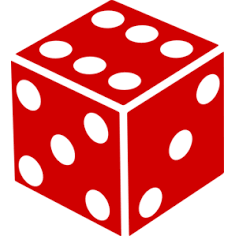Figure 4.19: A single die ​​

Example

The odds for rolling a single die and getting a two can be expressed as 1:5 (expressed “1 to 5”), meaning that in the long run, you would expect to get one event of a two for every five times there is an event of something other than a two. To extend this, if you were to roll a die, say, 600 times, you would expect to get 100 rolls of a two and 500 rolls of something other than a two. That gives us a ratio of 100 to 500, reduced to 1:5. Odds against just reverses this.

The confusion arises when the odds ratio is mistakenly taken to represent a probability that one out of five rolls will result in a two, making the probability .20 instead of the correct probability of one out of six, or .1667.

4.22

An online survey has boosted participation by offering a prize to every 20th participant who completes a survey. How would the likelihood of receiving a prize be stated as odds?

A

1:19

B

1:20

C

1:21

D

.05

4.23

In the previous question, what is the probability of receiving a prize?

4.24

Odds represent a ratio of $\_\_\_\_\_\_\_$ to non-events.

## Case Study: The Monty Hall ProblemFigure 4.20: Monty Hall, the host of Let's Make a Deal.  ​

If you still need convincing that probability problems have a place in real life, consider the game show Let's Make a Deal.

Contestants were given a choice on the show to select one of three doors. One door conceals a new car, while the other two conceal goats.

Once they chose, the host of the show, Monty Hall, opened one of the other two doors that contained a goat. At that point, the contestant knows there is a goat behind one of the doors Monty did not select.

Then Monty Hall asks the contestant, “Do you want to switch doors?”

Case Study Question 4.01

What is the original probability of Door I concealing the new car?

A

1/3

B

2/3

C

3/3

D

1/4

Case Study Question 4.02

Did the probability of Door I concealing the car change when Monty Hall revealed the goat behind Door II?

A

Yes

B

No

Want to try the Monty Hall Problem for yourself? Click here to try a simulation.

Case Study Question 4.03

The dilemma now is whether to switch doors or stay with the original choice. What should the contestant do? Is it to their advantage to stay or switch doors?

A

Stay

B

Switch

Watch below for an explanation of the probabilities behind the Monty Hall Problem.

## Pre-Class Discussion Questions

### Class Discussion 4.01

Class Discussion 4.01

What do we mean by “probability”? I.e., what is it, exactly?

Click here to see the answer to Class Discussion 4.01.

### Class Discussion 4.02

Class Discussion 4.02

Describe a probability experiment and give an example.

Click here to see the answer to Class Discussion 4.02.

### Class Discussion 4.03

Class Discussion 4.03

What is sample space, and what is its role in a probability experiment?

Click here to see the answer to Class Discussion 4.03.

### Class Discussion 4.04

Class Discussion 4.04

What are the types of probability?

Click here to see the answer to Class Discussion 4.04.

### Class Discussion 4.05

Class Discussion 4.05

Distinguish between the multiplication rule and the addition rule of probability.

Click here to see the answer to Class Discussion 4.05.This Content is Locked
Only a limited preview of this text is available. You'll need to sign up to Top Hat, and be a verified professor to have full access to view and teach with the content.

## Answers to Pre-Class Discussion Questions

### Answer to Class Discussion 4.01

Probability is the numerical expression of the likelihood of an event, ranging from 0 to 1, where 0 indicates the event can never happen and 1 indicates it must happen. It can be summarized as 0 ≤ P(E) ≤ 1, where P(E) represents the probability of event E

Click here to return to Class Discussion 4.01.

### Answer to Class Discussion 4.02

A probability experiment can be any situation involving chance that leads to an observable and measurable outcome. All probability experiments:

• must have more than one possible outcome,
• have outcomes that can be specified in advance, and
• have outcomes that are due to chance.

A simple example: tossing a coin to see if tails comes up.

Click here to return to Class Discussion 4.02.

### Answer to Class Discussion 4.03

A sample space summarizes and describes all possible outcomes of a probability experiment. It is often presented in graph or tabular format.

Click here to return to Class Discussion 4.03.

### Answer to Class Discussion 4.04

There are three basic types of probability, each with defining characteristics:

• Theoretical probability is based on a theoretical assumption about the probability of the event.
• Empirical probability is based on observation of actual outcomes.
• Subjective probability is based on personal beliefs and may differ from other individual’s subjective probability of the same event.

Click here to return to Class Discussion 4.04.

### Answer to Class Discussion 4.05

The multiplication rule gives the probability of two (or more) events occurring in sequence, i.e., the probability of event A and event B.

The addition rule gives the probability that one or the other of two events will occur, i.e., the probability of event A or event B.

Click here to return to Class Discussion 4.05.

## Image Credits

 Image courtesy of the John Oxley Library, State Library of Queensland in the public domain.

 Image courtesy of ABC Television in the public domain.